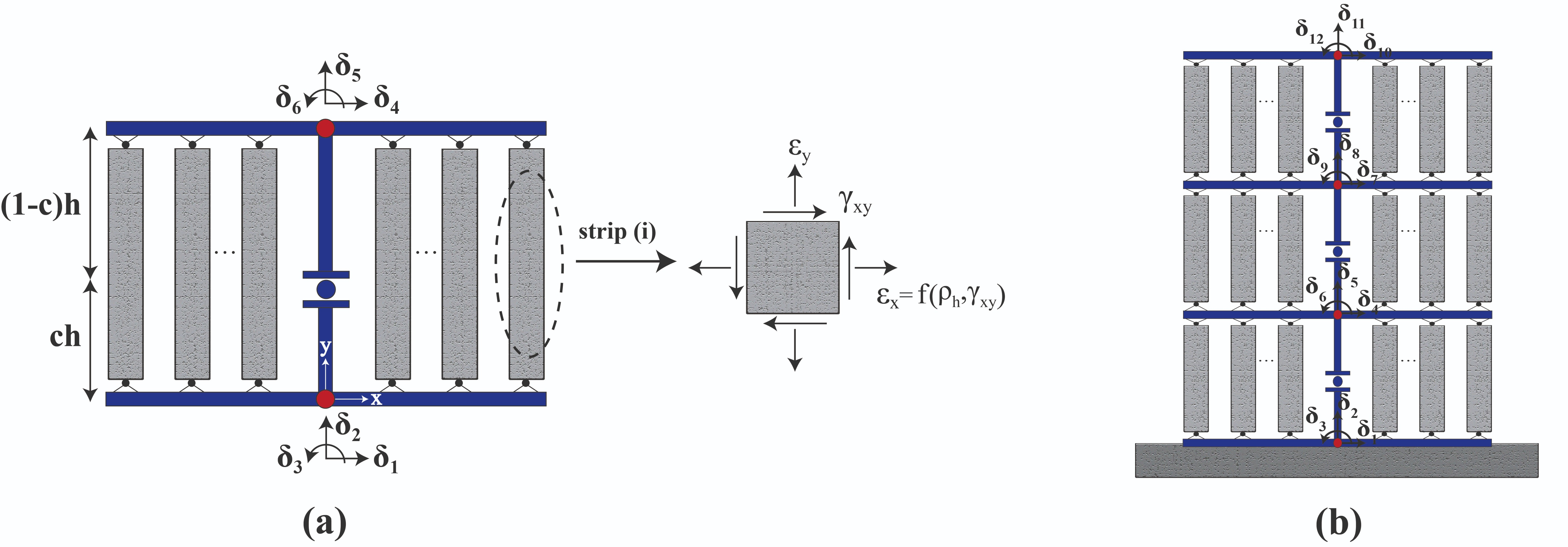# 3.1.9.10. E-SFI Element¶

The Efficient Shear-Flexure Interaction (E-SFI) element was developed based on the SFI-MVLEM formulation. The E-SFI element incorporates the shear-flexure interaction phenomenon by replacing the $$m$$ number of uniaxial fibers of the MVLEM, by two-dimensional RC panel elements subjected to membrane actions (FSAM). An E-SFI element is described by six degrees of freedom, and therefore no additional degrees of freedom are incorporated into the original MVLEM formulation, as in the SFI-MVLEM. The curvature of an E-SFI element is assumed to be uniform, and the resultant rotation is concentrated at height $$ch$$. The kinematic assumption of plane sections remain plane, as well as the assumption of constant shear strain along the element length, are considered for computing the axial and shear strains for each panel over the entire section. To complete the strain field of a panel element, a calibrated expression for the horizontal normal strain $$\varepsilon_{x}$$ is implemented to obtain accurate predictions from squat to slender RC walls.Fig. 3.1.9.10 E-SFI Element: (a) Element idealization; (b) Wall model.

This command is used to construct an E-SFI element object.

Command

element E_SFI $eleTag$iNode $jNode$m $c -thick$thicknesses -width $widths -mat$matTags

Parameter

Type

Description

$eleTag integer unique element object tag$iNode $jNode 2 integer tags of element nodes defined in upward direction$m

integer

number of element macro-fibers

$c float location of center of rotation from the base (c = 0.4 - recommended)$thicknesses

list float

a list of m macro-fiber thicknesses

$widths list float a list of m macro-fiber widths$matTags

list int

a list of m macro-fiber nDMaterial tags

The following recorders are available with the E-SFI element.

Recorder

Description

globalForce

element global forces

Curvature

element curvature

ShearDef

element deformation

RCPanel $fibTag$Response

returns RC panel (macro-fiber) $Response for a$fibTag-th panel (1 ≤ fibTag ≤ m). For available \$Response(s) refer to nDMaterial

Notes

1. This element shall be used in domain defined with -ndm 2 -ndf 3

Examples

The following example constructs an E-SFI element with tag 1 between nodes 1 and 2, with 8 macro-fibers and a location of center of rotation of 0.4. Each macro-fiber has a thickness 1, width 1 and nDMaterial of tag 1.

1. Tcl Code

element E_SFI 1 1 2 8 0.4 -thick 1 1 1 1 1 1 1 1 -width 1 1 1 1 1 1 1 1 -mat 1 1 1 1 1 1 1 1;

1. Python Code

element('E_SFI', 1, 1, 2, 8, 0.4, '-thick', 1, 1, 1, 1, 1, 1, 1, 1, '-width', 1, 1, 1, 1, 1, 1, 1, 1, '-mat', 1, 1, 1, 1, 1, 1, 1, 1)


REFERENCES:

1. Massone, L. M., López, C. N., & Kolozvari, K. (2021). Formulation of an efficient shear-flexure interaction model for planar reinforced concrete walls. Engineering Structures, 243, 112680. (link).

2. López, C. N., Massone, L. M., & Kolozvari, K. (2022). Validation of an efficient shear-flexure interaction model for planar reinforced concrete walls. Engineering Structures, 252, 113590. (link).

3. López C. N. Efficient shear-flexure interaction model for nonlinear analysis of reinforced concrete structural walls. MS Dissertation. Santiago, Chile: University of Chile; 2021. (link).

Code Developed by: C. N. López (University of Chile), L. M. Massone (University of Chile), K. Kolozvari (CSU Fullerton).